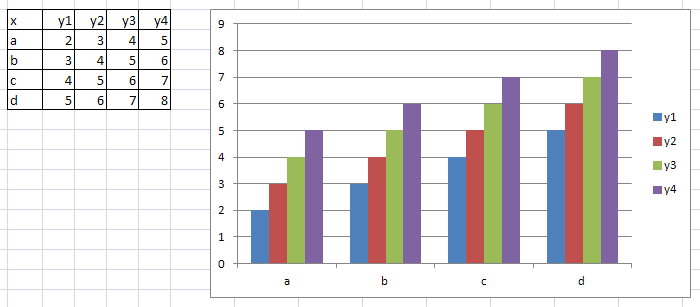## vbar multiple analysis vars

hi, i thought this would be easy but i cannot find a way to do it (in a simple way in sas913).

i want to create a bar chart with multiple analysis vars, like the chart below i created in excel:how can i do this in sas?

thanks, Bart

4 REPLIES 4art297
Opal | Level 21

## Re: vbar multiple analysis vars

SAS graph is definitely NOT my forte, but the following gets a fairly close result to what you want:

data have;

input x \$ y1-y4;

cards;

a 2 3 4 5

b 3 4 5 6

c 4 5 6 7

d 5 6 7 8

;

data need (keep=x time y);

set have;

array thedata(*) y1-y4;

do time=1 to dim(thedata);

y=thedata(time);

output;

end;

run;

proc gchart data=need;

vbar y / discrete

type=sum sumvar=y

group=x

space=0

gspace=4

subgroup=time /* this controls the coloring */

maxis=axis1 /* midpoint axis */

raxis=axis2 /* response/numeric axis */

gaxis=axis3 /* group axis */

autoref /* reflines at every major axis tickmark */

clipref /* put reflines behind the bars */

cref=graycc

legend=legend1

coutline=black

des=""

run;

quit;

## Re: vbar multiple analysis vars

thanks i will give this a go.Jay54
Meteorite | Level 14

## Re: vbar multiple analysis vars

Using Art's data, reshape the data by groups and use proc GCHART.

data group;
keep x grp val;
set have;
grp='Y1'; val=y1; output;
grp='Y2'; val=y2; output;
grp='Y3'; val=y3; output;
grp='Y4'; val=y4; output;
run;

proc gchart data=group;

vbar grp / sumvar=val group=x subgroup=grp autoref clipref;

run;

quit;

## Re: vbar multiple analysis vars

And one more variation ...

data my_data;
input groupvar \$ y1-y4;
cards;
a 2 3 4 5
b 3 4 5 6
c 4 5 6 7
d 5 6 7 8
;
run;

proc transpose data=my_data out=tran_data;
by groupvar;
run;

data tran_data; set tran_data (rename=(col1=value _name_=category));
label category='Category';
run;

legend1 label=none across=1 position=(right middle) shape=bar(.15in,.15in)
offset=(-10,0);

axis1 label=none minor=none order=(0 to 9 by 1);
axis2 label=none value=none;
axis3 label=none;

pattern1 v=s c=cx4f81bd;
pattern2 v=s c=cxc0504d;
pattern3 v=s c=cx9bbb59;
pattern4 v=s c=cx8064a2;

proc gchart data=tran_data;
vbar category / type=sum sumvar=value
group=groupvar
subgroup=category
space=0
legend=legend1
raxis=axis1
maxis=axis2
gaxis=axis3
noframe
autoref cref=graydd clipref
coutline=same
;
run;

Discussion stats
• 4 replies
• 863 views
• 3 likes
• 4 in conversation